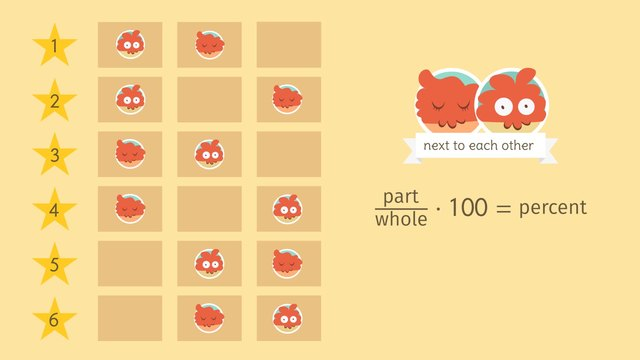# Counting ProblemsRate this video

Ø 5.0 / 1 ratings

The authorMatt W.

## Information about the videoCounting Problems

After this lesson, you will be able to compute percentages by using the formula, (Part/Whole) x 100 = Percent.

The lesson begins by teaching you how to identify the numerical values for the terms part and whole from a given context. It leads you to learn the formula, (Part/Whole) x 100 = Percent. It concludes with solving counting problems.

Learn how to compute percentages by helping Terence with his counting problems.

This video includes key concepts, notation, and vocabulary such as the terms whole and part that are used to find the percent in the formula, (Part/Whole) x 100 = Percent. Whole represents the total number of outcomes and part represents the number of outcomes that fit the criteria you’re looking for.

Before watching this video, you should already be familiar with solving the equation Quantity = Percent x Whole for one of the missing values, converting percents into fractions and decimals (and the other way around), and computations with fractions.